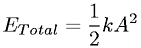Equations > Physics > Oscillations and Waves > Total energy of simple harmonic motion

### Total energy of simple harmonic motionLatex Code:

MathML Code:

 ${E}_{\mathrm{Total}}=\frac12{\mathrm{kA}}^{2}$

MathType 5.0: